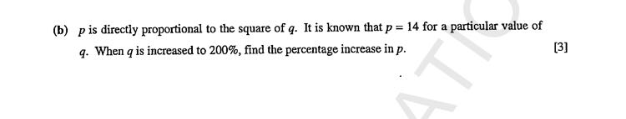# QuestionHi, can anyone help me with this question? Thanks!

We shall use a table to do this.

p           14       p

Q            q       2q

Q2        q2      4q2

By proportion

p = kq2

K = p/q2

So

14/q2  = p / 4q2

P = 14× 4 = 56

56/14 ×100% = 400%

400% – 100% = 300% increase.

Thus the answer is 300% increase.